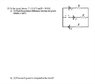# Potential Difference and Power in Resistor Circuit

• Gwozdzilla

## Homework Statement

I attached a screenshot of the problem(s) that my professor gave in a practice exam.

## Homework Equations

∑I = 0 at each junction
∑V = 0 at each closed loop
P = I2R = V2/R
Rseries = R1 + R2 +...

## The Attempt at a Solution

Part A:
I think the current I in the center, broken line of current, is zero because that wire is broken. Then
I1+I2 = 0. So I1 = I2 = I

Going around the large loop, starting from the battery at the top:

V + V - IR - IR = 0
2V = 2IR
V=IR
12 = I(10)
I = 1.2

In the bottom loop...

Va + V - IR + V = Vb
Va - Vb = -2V + IR
Va - Vb = -2(12) + (1.2)(10)
Va - Vb = 12V in absolute value...
Is absolute value what I'm looking for? +12V is the answer in the solutions my professor provided.

Part B:
Rseries = R1 + R2 +...
Rseries = 10 + 10
Rseries = 20

P = I2R = V2/R
P = (1.2)2(20) = 28.8W
P = (12)2/(20) = 7.2W

These aren't equal. I tried it with R in parallel as well (Req = 5), and it's still wrong. In the answers provided each of the power equations are multiplied by 2 and R = 10. Why is all of this?

Thanks!

#### Attachments

•2006 Final.13.jpg
11.5 KB · Views: 376

## Homework Statement

I attached a screenshot of the problem(s) that my professor gave in a practice exam.

## Homework Equations

∑I = 0 at each junction
∑V = 0 at each closed loop
P = I2R = V2/R
Rseries = R1 + R2 +...

## The Attempt at a Solution

Part A:
I think the current I in the center, broken line of current, is zero because that wire is broken. Then
I1+I2 = 0. So I1 = I2 = I

Going around the large loop, starting from the battery at the top:

V + V - IR - IR = 0
2V = 2IR
V=IR
12 = I(10)
I = 1.2

In the bottom loop...

Va + V - IR + V = Vb
Va - Vb = -2V + IR
Va - Vb = -2(12) + (1.2)(10)
Va - Vb = 12V in absolute value...
Is absolute value what I'm looking for? +12V is the answer in the solutions my professor provided.

Va-Vb=-12 V, The potential increases from a to b by 12 V. Look at your notes, what Uab means, it might be Vb-Va.

Part B:
Rseries = R1 + R2 +...
Rseries = 10 + 10
Rseries = 20

P = I2R = V2/R
P = (1.2)2(20) = 28.8W
P = (12)2/(20) = 7.2W

The 28.8 V is correct. The voltage is 24 V across the two series resistors, so P=242/20, the same as before.

ehild